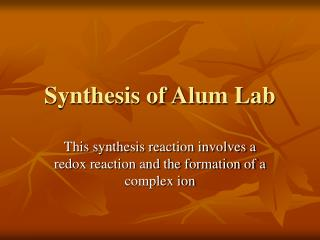DownloadDownload PresentationSynthesis of Alum Lab

# Synthesis of Alum Lab

Download Presentation## Synthesis of Alum Lab

- - - - - - - - - - - - - - - - - - - - - - - - - - - E N D - - - - - - - - - - - - - - - - - - - - - - - - - - -
##### Presentation Transcript

1. Synthesis of Alum Lab This synthesis reaction involves a redox reaction and the formation of a complex ion

2. Balanced Net Ionic Equations • Mole ratios of Al to K to alum are all 1:1. • Aluminum hydroxide dissolves in a solution with excess KOH: Al(OH)3(s) + OH-(aq) [Al(OH)4]-(aq) • Aluminum hydroxide dissolving in acid solution: Al(OH)3(s) + 3H+(aq) + 3H2O(l) [Al(H2O)63+]-(aq) + 3H2O(l)

3. Lab Discussion Questions • Aluminum reacts with KOH and water to form tetrahydroxoaluminate(III) ions, potassium ions and hydrogen gas: 2Al(s) + 2K+(aq) + 2OH-(aq) + 6H2O(l) 2[Al(OH)4]-(aq) + 2K+(aq) + 3H2(g) The oxidation and reduction half reactions: Oxidation: 2Al(s) + 8OH-(aq) + 6H2O(l) 2[Al(OH)4]-(aq) + 6e- Reduction: 6H2O(l)+ 6e- 3H2(g) + 6OH-(aq)

4. Hydrogen ions from the acid react with tetrahydroxoaluminate(III) ions to precipitate aluminum hydroxide: H+(aq) + [Al(OH)4]-(aq)  Al(OH)3(s) + H2O(l) c) Aluminum hydroxide reacts with additional hydrogen ions and water to form hydrated aluminum ions: Al(OH)3(s) + 3H2O(l) + 3H+(aq)  [Al(H2O)6]3+(aq) d) Alum forms from potassium ions, hydrated aluminum ions sulfate ions and water: K+(aq) + 6H2O(l) + 2SO42-(aq) + [Al(H2O)6]3+(aq) KAl(SO4)2.12H2O(s)

5. Meanings of These Formulae • A complex ion consists of a central metal cation (usually derived from a transition metal) joined by coordinate covalent bonds to molecules or anions called ligands. Complex ions can be cations or anions. • Compounds composed of a complex ion and counter ions are coordinate compounds • Hydrates are indicated by the dot and number of water molecules incorporated into the compound. When finding molar mass of a hydrate, add the indicated number of water molecules (12H2O = (12 x 18 g/mol).

6. Relationship between coordinate compound and complex ion

7. Common complex ions • The number of ligands (things attached to the metal) is usually double the oxidation number of the metal. Transition metals with NH3, OH-, or SCN- often form complexes. • NH3 is added to Cu2+: Cu(NH3)42+ cupric tetraamine • NH3 is added to Ag+: Ag(NH3)2+ silver diamine • Conc. OH- is added to Zn(OH)2: Zn(OH)42+ • Fe3+ in thiocyanate (SCN-): Fe(SCN)63- complex • Co2+ with chlorine: CoCl42- • Al oxidized in base: Al(OH)4-

8. Counting the Charges

9. Naming Coordination Complexes • Cation name precedes anion name • The complex ion: • Ligands are listed first then central metal ion • Negative ligands are often named by replacing the ion ending with an –o. (chloro, bromo, nitrito, etc.). • Examples of ligand names are chloro, hydroxo, cyano • Ligand Names Not Ending in –o: H2O - aqua NH3 - ammine CH3NH2 - methylamine CO - carbonyl NO – nitrosyl S2O32--- thiosulfato

10. WRITING COMPLEX ION FORMULAS • write the central ion first • then negative ligands (alphabetical order) • then neutral molecules (alphabetical order) • polyatomics always in parentheses (even if 1)